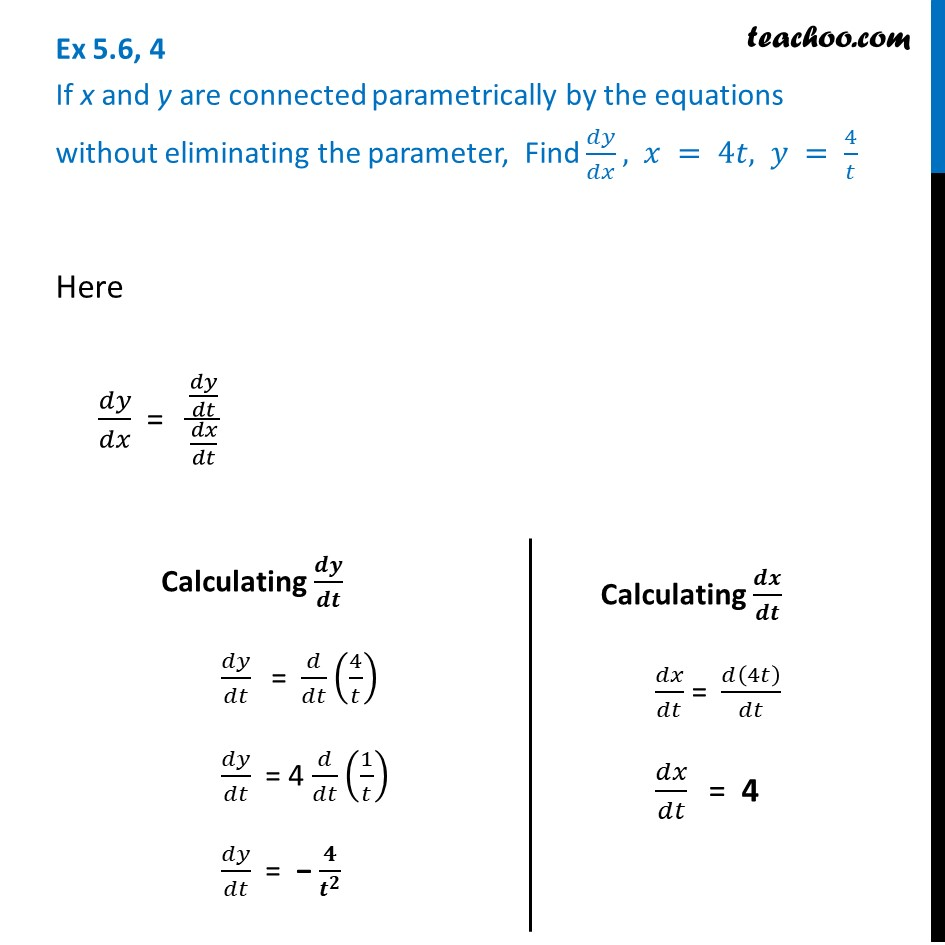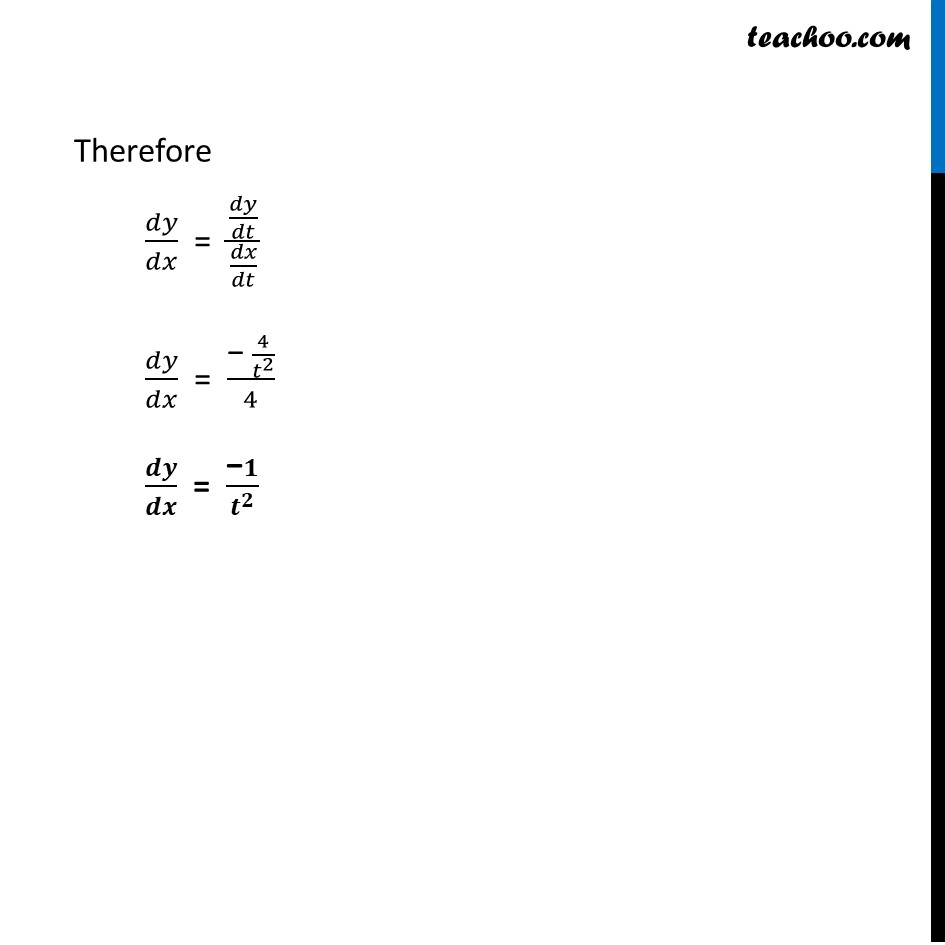Ex 5.6

Chapter 5 Class 12 Continuity and Differentiability
Serial order wiseLearn in your speed, with individual attention - Teachoo Maths 1-on-1 Class

### Transcript

Ex 5.6, 4 If x and y are connected parametrically by the equations without eliminating the parameter, Find 𝑑𝑦/𝑑𝑥, 𝑥 = 4𝑡, 𝑦 = 4/𝑡Here 𝑑𝑦/𝑑𝑥 = (𝑑𝑦/𝑑𝑡)/(𝑑𝑥/𝑑𝑡) Calculating 𝒅𝒚/𝒅𝒕 𝑑𝑦/𝑑𝑡 " " = 𝑑/𝑑𝑡 (4/𝑡) 𝑑𝑦/𝑑𝑡 = 4 𝑑/𝑑𝑡 (1/𝑡) 𝑑𝑦/𝑑𝑡 = − 𝟒/𝒕^𝟐 Calculating 𝒅𝒙/𝒅𝒕 𝑑𝑥/𝑑𝑡 = 𝑑(4𝑡)/𝑑𝑡 𝑑𝑥/𝑑𝑡 " " = 4 Therefore 𝑑𝑦/𝑑𝑥 = (𝑑𝑦/𝑑𝑡)/(𝑑𝑥/𝑑𝑡) 𝑑𝑦/𝑑𝑥 = ("− " 4/𝑡^2 )/4 𝒅𝒚/𝒅𝒙 = ("−" 𝟏)/𝒕^𝟐